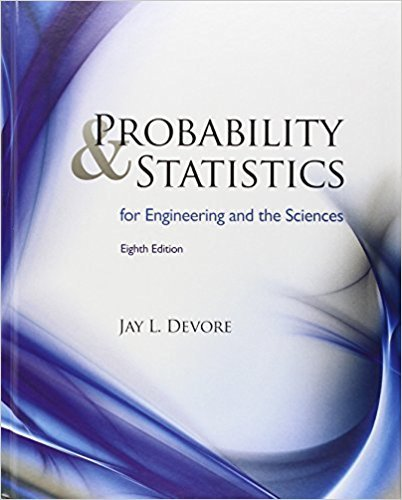×
Get Full Access to Statistics - Textbook Survival Guide
Get Full Access to Statistics - Textbook Survival Guide

×

Textbooks / Statistics / Probability and Statistics for Engineering and the Sciences 8

# Probability and Statistics for Engineering and the Sciences 8th Edition - Solutions by Chapter## Full solutions for Probability and Statistics for Engineering and the Sciences | 8th Edition

ISBN: 9780538733526Probability and Statistics for Engineering and the Sciences | 8th Edition - Solutions by Chapter

Solutions by Chapter
4 5 0 335 Reviews
##### ISBN: 9780538733526

Probability and Statistics for Engineering and the Sciences was written by and is associated to the ISBN: 9780538733526. This textbook survival guide was created for the textbook: Probability and Statistics for Engineering and the Sciences , edition: 8. Since problems from 16 chapters in Probability and Statistics for Engineering and the Sciences have been answered, more than 89579 students have viewed full step-by-step answer. This expansive textbook survival guide covers the following chapters: 16. The full step-by-step solution to problem in Probability and Statistics for Engineering and the Sciences were answered by , our top Statistics solution expert on 08/08/17, 06:52AM.

Key Statistics Terms and definitions covered in this textbook
• Acceptance region

In hypothesis testing, a region in the sample space of the test statistic such that if the test statistic falls within it, the null hypothesis cannot be rejected. This terminology is used because rejection of H0 is always a strong conclusion and acceptance of H0 is generally a weak conclusion

• Alias

In a fractional factorial experiment when certain factor effects cannot be estimated uniquely, they are said to be aliased.

• Arithmetic mean

The arithmetic mean of a set of numbers x1 , x2 ,…, xn is their sum divided by the number of observations, or ( / )1 1 n xi t n ? = . The arithmetic mean is usually denoted by x , and is often called the average

• Binomial random variable

A discrete random variable that equals the number of successes in a ixed number of Bernoulli trials.

• Cause-and-effect diagram

A chart used to organize the various potential causes of a problem. Also called a ishbone diagram.

• Completely randomized design (or experiment)

A type of experimental design in which the treatments or design factors are assigned to the experimental units in a random manner. In designed experiments, a completely randomized design results from running all of the treatment combinations in random order.

• Conditional probability distribution

The distribution of a random variable given that the random experiment produces an outcome in an event. The given event might specify values for one or more other random variables

• Conidence interval

If it is possible to write a probability statement of the form PL U ( ) ? ? ? ? = ?1 where L and U are functions of only the sample data and ? is a parameter, then the interval between L and U is called a conidence interval (or a 100 1( )% ? ? conidence interval). The interpretation is that a statement that the parameter ? lies in this interval will be true 100 1( )% ? ? of the times that such a statement is made

• Contingency table.

A tabular arrangement expressing the assignment of members of a data set according to two or more categories or classiication criteria

• Continuous distribution

A probability distribution for a continuous random variable.

• Control chart

A graphical display used to monitor a process. It usually consists of a horizontal center line corresponding to the in-control value of the parameter that is being monitored and lower and upper control limits. The control limits are determined by statistical criteria and are not arbitrary, nor are they related to speciication limits. If sample points fall within the control limits, the process is said to be in-control, or free from assignable causes. Points beyond the control limits indicate an out-of-control process; that is, assignable causes are likely present. This signals the need to ind and remove the assignable causes.

• Counting techniques

Formulas used to determine the number of elements in sample spaces and events.

• Cumulative distribution function

For a random variable X, the function of X deined as PX x ( ) ? that is used to specify the probability distribution.

• Cumulative sum control chart (CUSUM)

A control chart in which the point plotted at time t is the sum of the measured deviations from target for all statistics up to time t

• Dispersion

The amount of variability exhibited by data

• Error sum of squares

In analysis of variance, this is the portion of total variability that is due to the random component in the data. It is usually based on replication of observations at certain treatment combinations in the experiment. It is sometimes called the residual sum of squares, although this is really a better term to use only when the sum of squares is based on the remnants of a model-itting process and not on replication.

• Expected value

The expected value of a random variable X is its long-term average or mean value. In the continuous case, the expected value of X is E X xf x dx ( ) = ?? ( ) ? ? where f ( ) x is the density function of the random variable X.

• Fisher’s least signiicant difference (LSD) method

A series of pair-wise hypothesis tests of treatment means in an experiment to determine which means differ.

• Fraction defective

In statistical quality control, that portion of a number of units or the output of a process that is defective.

• Harmonic mean

The harmonic mean of a set of data values is the reciprocal of the arithmetic mean of the reciprocals of the data values; that is, h n x i n i = ? ? ? ? ? = ? ? 1 1 1 1 g .